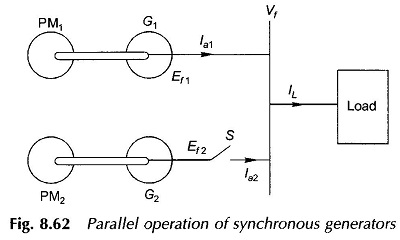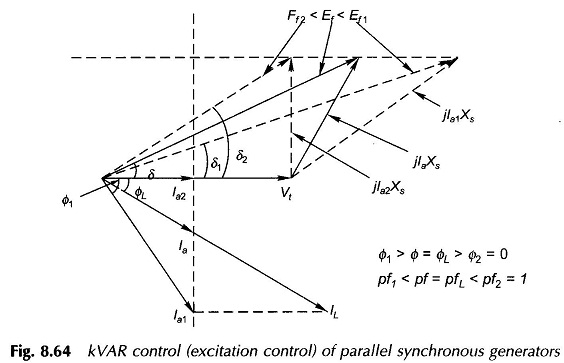# Parallel Operation of Synchronous Generators

## Parallel Operation of Synchronous Generators:

Parallel Operation of Synchronous Generators – The operation considered in Sec. 8.8 is that of a synchronous machine connected to infinite bus-bars. Here the parallel operation of two finite size synchronous generators will be considered, which is the way large practical size generators are used. In a power system the generators are connected to the nodes of a grid composed of a network of transformers and transmission lines. A national level grid* may comprise even hundreds of generators and hundreds of kilometres of the transmission line. The grid formation is dictated by reasons of reliability (continuity of supply) and by investment and operating economics of the power plant.

Figure 8.62 shows two synchronous generators along with their primemovers to be operated in parallel. After the two generators are brought to their respective synchronous speeds and their field currents adjusted to give nearly equal terminal voltages, switch S is closed in accordance with the synchronizing procedure described in Sec. 8.7. Active and reactive powers, supplied to the common load by each generator, are controlled respectively by their primemover throttles and field currents as was observed in Sec. 8.8.

The active power-sharing between paralleled generators is dependent upon thedroop of the frequency (speed)-power characteristics of the primemovers and their governors. Theses characteristics are nearly linear for small changes in the operating (rated) frequency and power as shown in Fig. 8.63. These characteristics can be slided up or down by adjustment of the set points of their governors. Drooping* frequency-power characteristics are essential for proper loading between generators and the adjustment thereof.Changes in excitation of parallel-operating generators affect the terminal voltage and reactive-power loading with active power sharing remaining unchanged (primemover governor settings are not disturbed). This was clarified with respect to a single machine connected to infinite bus-bars in Sec. 8.8, Fig. 8.32(a). For the system of two generators as in Fig. 8.62, assume that the two generators are identical, their primemover governors are adjusted for equal load sharing and their excitations are equal; so that they operate with identical currents and power factors (i.e. both active and reactive powers are equally shared). The phasor diagram under these operating conditions, identical for each generator, is shown in Fig. 8.64 in thick line.

For the given total load and equal sharing of real load, let the excitation of G1 be now increased and that of G2 simultaneously reduced in such a manner as to keep the terminal voltage unchanged. Each generator, therefore, behaves as if it is connected to infinite bus-bars, i.e., Ef sin S will remain constant. With active power on each generator fixed (at half the total), the projection of generator (each) current on V. (active current component) will also remain constant and as a result the power factor of G1 goes down and that of G2 improves (figure is drawn for upf). Thus G1 supplies more kVAR to load and G2 supplies an equal amount less. This is how reactive-load sharing between generators can be controlled. If, however, the excitation of only one of the generators is raised (or lowered), the terminal voltage will increase (or decrease) while this generator shares a larger (or smaller) part of load kVAR. For raising (lowering) the terminal voltage without modifying the kVAR sharing, the excitation of both the generators must be raised (or lowered). Excitation is automaticallycontrolled by voltage regulators acting on the field circuits of generators. All that is needed to be done is to change the set-points of voltage regulators.

The primemover governor and generator voltage regulator control loops are assumed independent in the above. They are, however, weakly coupled because of losses in generators. Independence of control loops is a good working assumption.

Updated: April 18, 2018 — 11:20 am

`Please enable JavaScript in your browser to complete this form.Name *FirstLastEmail *Paragraph TextName`
` `